Test: Graph Theory

# Test: Graph Theory

Test Description

## 10 Questions MCQ Test GATE Electrical Engineering (EE) 2023 Mock Test Series | Test: Graph Theory

Test: Graph Theory for Railways 2023 is part of GATE Electrical Engineering (EE) 2023 Mock Test Series preparation. The Test: Graph Theory questions and answers have been prepared according to the Railways exam syllabus.The Test: Graph Theory MCQs are made for Railways 2023 Exam. Find important definitions, questions, notes, meanings, examples, exercises, MCQs and online tests for Test: Graph Theory below.
Solutions of Test: Graph Theory questions in English are available as part of our GATE Electrical Engineering (EE) 2023 Mock Test Series for Railways & Test: Graph Theory solutions in Hindi for GATE Electrical Engineering (EE) 2023 Mock Test Series course. Download more important topics, notes, lectures and mock test series for Railways Exam by signing up for free. Attempt Test: Graph Theory | 10 questions in 30 minutes | Mock test for Railways preparation | Free important questions MCQ to study GATE Electrical Engineering (EE) 2023 Mock Test Series for Railways Exam | Download free PDF with solutions
 1 Crore+ students have signed up on EduRev. Have you?
Test: Graph Theory - Question 1

### Consider the following circuits :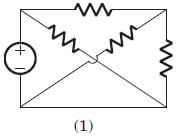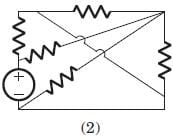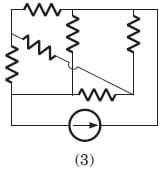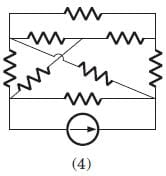The planner circuits are

Detailed Solution for Test: Graph Theory - Question 1

The circuit 1 and 2 are redrawn as below. 3 and 4 can not be redrawn on a plane without crossing other branch.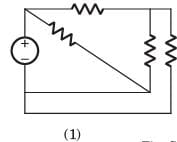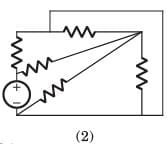Test: Graph Theory - Question 2

### Consider the following graphs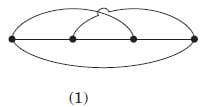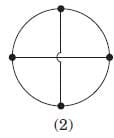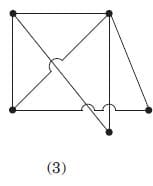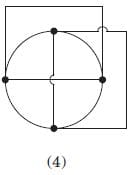Non-planner graphs are

Detailed Solution for Test: Graph Theory - Question 2

Other three circuits can be drawn on plane without crossing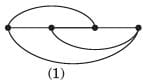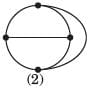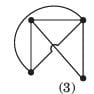Test: Graph Theory - Question 3

### A graph of an electrical network has 4 nodes and 7 branches. The number of links l, with respect to the chosen tree, would be

Detailed Solution for Test: Graph Theory - Question 3

l = b - (n - 1) = 4.

Test: Graph Theory - Question 4

For the graph shown in fig.correct set is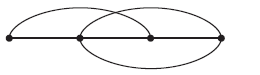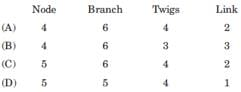Detailed Solution for Test: Graph Theory - Question 4

There are 4 node and 6 branches.

t = n - 1 = 3, l = b - n + 1 = 3

Test: Graph Theory - Question 5

A tree of the graph shown in fig. is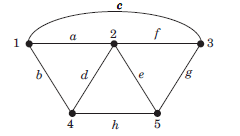Detailed Solution for Test: Graph Theory - Question 5

From fig. it can be seen that a f h g is a tree of given graph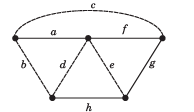Test: Graph Theory - Question 6

Branch current and loop current relation are expressed in matrix form as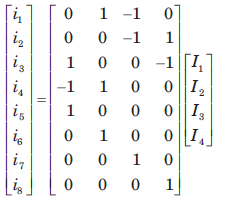where ij represent branch current and Ik loop current. The number of independent node equation are

Detailed Solution for Test: Graph Theory - Question 6

Number of branch = 8

Number of twigs = 8 - 4 = 4

Number of twigs = number of independent node equation.

Test: Graph Theory - Question 7

If the number of branch in a network is b, thenumber of nodes is n and the number of dependent loop is l, then the number of independent node equations will be

Detailed Solution for Test: Graph Theory - Question 7

The number of independent node equation are n - 1.

Test: Graph Theory - Question 8

Branch current and loop current relation are expressed in matrix form as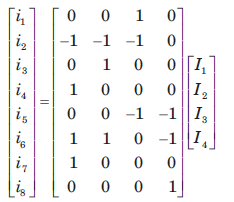.

where ij represent branch current and Ik loop current.

The rank of incidence matrix is

Detailed Solution for Test: Graph Theory - Question 8

Number of branch b = 8

Number of link l = 4

Number of twigs t = b - l = 4

rank of matrix = n - 1 = t = 4

Test: Graph Theory - Question 9

A network has 8 nodes and 5 independent loops. The number of branches in the network is

Detailed Solution for Test: Graph Theory - Question 9

l = b - (n - 1)

= 5 = b - 7

b = 12

Test: Graph Theory - Question 10

A branch has 6 node and 9 branch. The independent loops are

Detailed Solution for Test: Graph Theory - Question 10

l = b - (n-1) = 4

## GATE Electrical Engineering (EE) 2023 Mock Test Series

22 docs|274 tests
Information about Test: Graph Theory Page
In this test you can find the Exam questions for Test: Graph Theory solved & explained in the simplest way possible. Besides giving Questions and answers for Test: Graph Theory, EduRev gives you an ample number of Online tests for practice

## GATE Electrical Engineering (EE) 2023 Mock Test Series

22 docs|274 tests(Scan QR code)